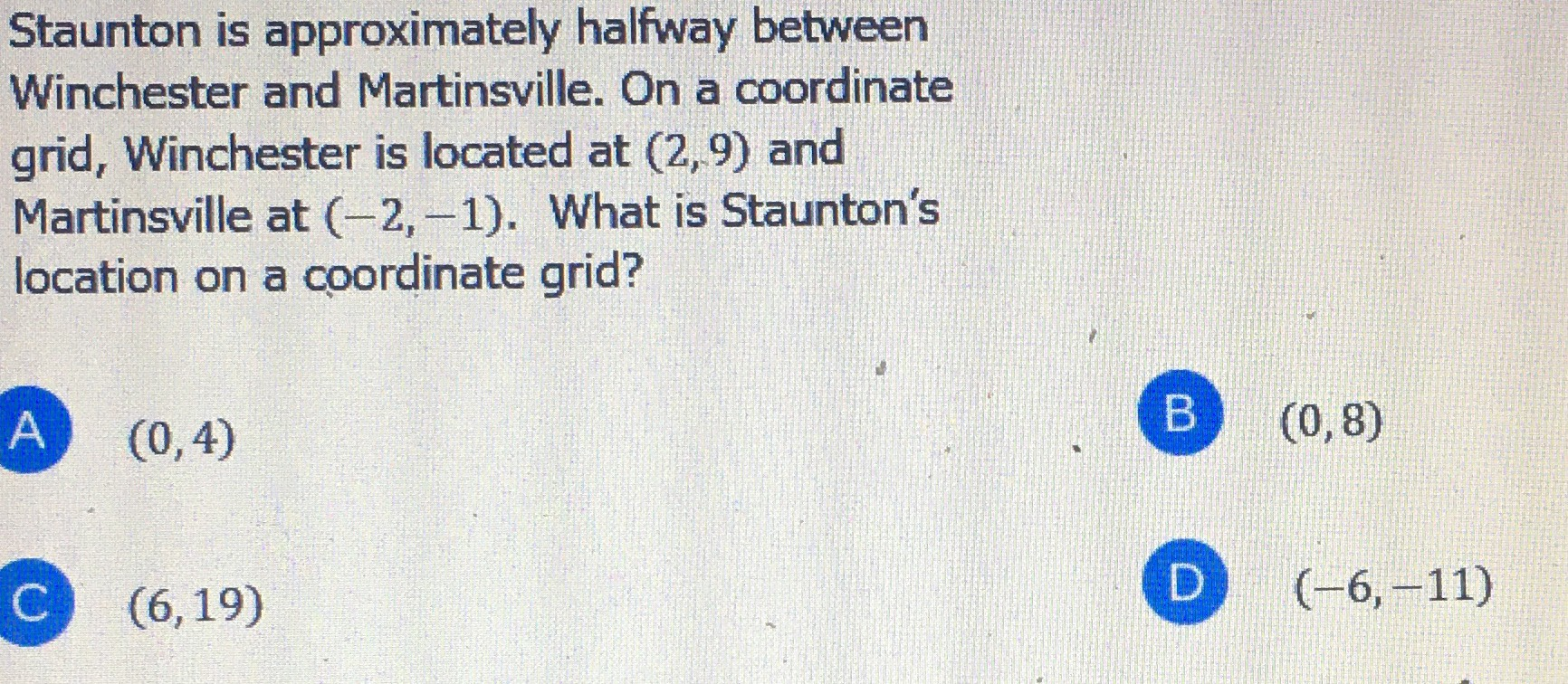### Still have math questions?

Algebra
QuestionStaunton is approximately halfway between Winchester and Martinsville. On a coordinate grid, Winchester is located at $$( 2,9 )$$ and Martinsville at $$( - 2 , - 1 )$$ . What is Staunton's location on a coordinate grid?

(A) $$( 0,4 )$$ (B) $$( 0,8 )$$ (C) $$( 6,19 )$$ (D) $$( - 6 , - 11 )$$